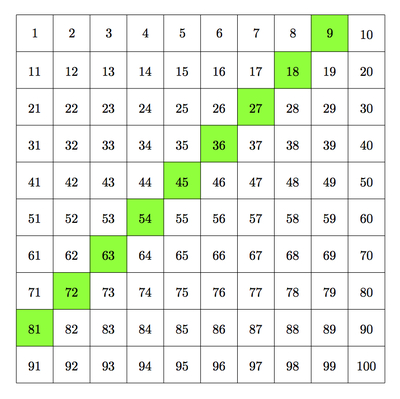# Multiples of nine

Alignments to Content Standards: 4.OA.C.5

1. Starting with 9, list the first 10 multiples of 9.
2. In the list in part (a) what patterns do you see with the digits in the 10's place? What patterns do you see with the digits in the 1's place?
3. Using pictures, words, or equations, explain the patterns you observed in part (b).

## IM Commentary

This task examines the interesting fact that when a number is divisible by 9 then the sum of its digits is also divisible by 9. Only one and two digit numbers are examined here. A natural extension of this activity would be to see if this pattern remains true for three digit or larger numbers. At this age, students are multiplying large numbers by a single digit number (4.NBT.5) and so they could be encouraged to pursue further this interesting fact about the sum of the digits in numbers divisible by 9. Understanding this rule provides excellent practice in place value and properties of arithmetic.

Three solutions are provided for part (c), explaining the pattern. One gives a verbal description, the second uses arithmetic manipulations, while the third uses a picture. Students working on this task will look for and make use of structure (MP7): in parts (b) and (c), students are looking both to identify a pattern and explain why the pattern holds. Students will also look for and express regularity in repeated reasoning (MP8), especially in part (c); patterns in general require identifying a process which is repeated multiple times.

## Solutions

Solution: 1 Arithmetic Properties

1. The first ten multiples of 9 are $$9, 18, 27, 36, 45, 54, 63, 72, 81, 90.$$

2. For the tens places, note that the number 9 can be thought of as 0 tens and 9 ones so the tens place has a 0. The tens place of 18 is 1. Going through the list of multiples of 9, the tens digits are $$0, 1, 2, 3, 4, 5, 6, 7, 8, 9.$$ For the ones place, we see that 9 has 9 ones, 18 has 8 ones, and going through the list we get ones values of $$9, 8, 7, 6, 5, 4, 3, 2, 1, 0.$$ So the tens place starts at 0 and go up by 1 while the ones place starts at 9 and goes down by 1.

Another important pattern that relates the ones and tens places is that if we add up the digit in the tens place and the digit in the ones place, we get 9 for each of these numbers.

3. We will explain why the digit in the tens place goes up by 1 and the digit in the ones place goes down by 1.

• To go to the next multiple of 9, we add 9 each time.
• We know that 9 = 10−1. So adding 9 is like adding 10 and then subtracting 1.
• Adding 10 increases the the digit in the tens place by 1 (as long as it is not 9). Subtracting 1 decreases the the digit in the ones place by 1 (as long as it is not 0).

Solution: 2 Equations and picture for part (c)

Here we use the properties of arithmetic to help explain why the tens value increases by 1 and the ones value decreases by 1 when adding 9. We study the equation 18+9=27 as an example. We can rewrite 18=1×10+8 and 9 = 10 - 1 to get

\begin{align} 18+9 &=(1×10+8)+(1×10−1) \\ &=1×10+(8+1×10)−1\\ &=1×10+(1×10+8)−1\\ &=(1×10+1×10)+(8−1)\\ &=(1+1)×10+(8−1). \end{align}

We have written out every step to see which rules of arithmetic are being used. The first line is arithmetic, the second line uses the associative property of addition, the third line uses the commutative property of addition, the fourth line uses the associative property of addition, and the fifth line uses the distributive property of multiplication over addition. Notice that the very last line shows why the tens place has increased by one while the ones places has decreased by one after adding 9.

The arithmetic steps above can be visualized in the table below:Each multiple of 9 is highlighted in green. To move from one multiple of 9 to the next, in the table, we move down one (adding 10) and to the left one (subtracting 1). The sum of the digits is still the same because the digit in the tens place has increased by 1 while the digit in the ones place has decreased by 1.

Solution: 3 Picture for (c)

Here we give a visual representation for the pattern in the multiples of 9. We first look at what happens with 9+9:To make a ten we have moved one green block from the first 9 to the second 9: this gives us a ten and eight ones. So the tens place has increased by one and the ones place has decreased by one. We can see the same pattern going from 18 to 27 by adding 9:Here too we take away one from the 8 in 18 and add it to the 9 to make a 10. The final picture looks a little out of order with the 7 ones between the two tens but we can shift this around to put the two tens together. If we look at the set of equations in the second solution, the fact that the strips are switched around in order to have the tens together shows up when the commutative property of addition is used.

This pattern will remain until we reach 90. When we add 9 to 90 there are no ones to take from in order to add to the 9 and make a 10.